# 2017年广东专插本《高等数学》考试真题1.下列极限等式不正确的是

A.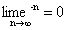B.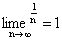C.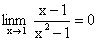C.2.若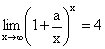，则常熟a=

A.㏑2 B.2㏑2 C.1 D.4

3.设F(x)是可导函数f(x)的一个原函数，C为任意常数，则下列不等式不正确的是

A.∫f’(x)dx=f(x)+C B.[∫f(x)dx]’=f(x)

C.∫f(x)dx=F(x)+C D.∫F(x)dx=f(x)+C

4.已知函数f(x)在区间[0,2]上连续，且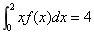，则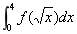=

A.2 B.4 C.6 D.8

5.将二次积分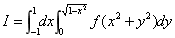化为极坐标形式的二次积分，则I=

A.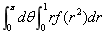B.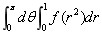C.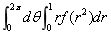D.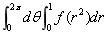6.已知当x→0是，f(x)～2x，则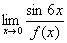= .

7.若常数p>1，则广义积分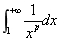=   .

8.设二元函数z=f(x,y)的全微分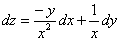，则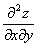=   .

9.微分方程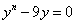的通解为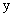=   .

10.级数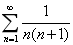的和为   .

11.求极限.

12.设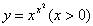，求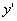.

13.设函数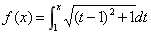，求曲线y=f(x)的凹凸区间和拐点.

14.求不定积分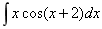.

15.设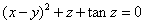，计算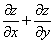.

16.求二重积分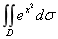，其中D是由曲线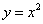和直线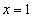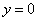围成的有界闭区域。

17.若曲线经过点(0,1)，且该曲线上任一点(x,y)处的切线斜率为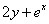，求这条直线.

18.判定级数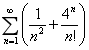的敛散性.

19.设函数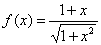.

（1）求曲线y=f(x)的水平渐近线方程；

（2）求由曲线y=f(x)和直线x=0，x=1及y=0围成的平面图形绕x轴旋转而成的旋转体体积V.

20.已知函数.

（1）证明：当x>0时，恒有（2）试问方程f(x)=x在区间(0,+∞)内有几个实根？